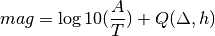# mb_IDC¶

Body wave magnitude computed at CTBTO/IDC (mb) is calculated for seismic events from the time-defining primary body waves recorded at seismic stations at an epicentral distance between 20 and 105 degrees from the event.

## Description¶

### Amplitude¶

The A5/2 amplitudes are calculated on the vertical component seismograms filtered between 0.8 and 4.5 Hz and converted to displacement.

### Station Magnitude¶with

A: amplitude of type A5/2

T: period of the signal in seconds

Q: attenuation correction function of event distance and event depth

h: event depth in km

The attenuation corrections as a function of distance and depth are based on (Veith, K. F., and Clawson, G. E., 1972). The corrections are tabulated every degree for distances out to 180 degrees and for depths 0, 15, 40 km, and 100-800 km in steps of 100 km. Bi-cubic splines were used for interpolating the tables. The tabulated values were adjusted for the fact that the original (Veith, K. F., and Clawson, G. E., 1972) tables relate to peak-to-peak amplitudes, whereas the measured amplitudes for mb calculations are half peak-to-peak. The default corrections are read from a file installed at @DATADIR@/magnitudes/IDC/qfvc.mb.If that file is not present no magnitude will be calculated.

### Station corrections¶

Station magnitudes can be computed with a station specific correction table which is configured in the global bindings. The parameter magnitudes.mb(IDC).Q takes a path and allows to use placeholders for network code ({net}), station code ({sta}) and location code ({loc}).

Example:

magnitudes.mb(IDC).Q = @DATADIR@/magnitudes/IDC/{net}.{sta}.mb

• Amplitude unit in SeisComP3: nanometer (nm)
• Time window: 5.5 s
• Default distance range: 20 - 105 deg
• Depth range: 0 - 800 km